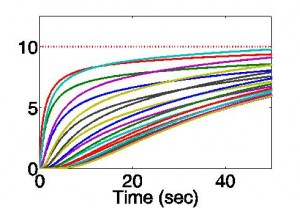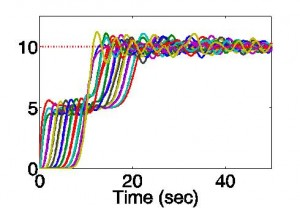# Proportional-Integral Distributed Optimization

Many tasks to be solved by multi-agent systems can be formed in terms of a cost, where task completion corresponds to minimizing the cost.  For example, the figure below depicts multi-agent formation control where agents must collectively decide upon parameters of the formation (such as displacement, rotation, and spread) while moving into position.  By having each agent impose a cost on distance to travel, the system of agents can choose the parameters that will result in the least distance traveled by the agents.  Distributed optimization techniques can then be employed to solve the task without any one agent being aware of the costs of the other agents.  In the Grits lab, we have developed a method for distributed optimization which combines two branches of distributed optimization methods under a proportional-integral control framework.

#### Solving a Constrained Optimization Problem

Distributed optimization is typically done by allowing each agent to maintain its own “version” of the variables being optimized.  Ideally, each agent would maintain the same value for the variables, and they would “slide” the variables down the constraint to arrive at the optimal value.  Below shows an example of two agents where one agent wants to have the value of the parameter to be 1 and the other agent wants the variable to be -1.  The constraint that they have the same version of the variable is shown as a red line.

However, computing the constrained gradient may be difficult to do in a distributed fashion as it may require the knowledge about every agent’s cost.  Thus, distributed optimization algorithms typically relax the constraint that every agent has the same value and add components to ensure that agents return back to the constraint at the optimal point, as shown below:

### Previous Distributed Optimization Methods

Two main camps for distributed optimization exist.  The first are consensus-based methods.  These methods consist of having agents perform a gradient step for optimization combined with a consensus step for agreement.  The other main category consists of dual-methods or dual-decomposition.  In this method, the optimization problem is solved by introducing the constraint that every agent have equal values and then solving the dual-problem.

Each method has its own benefits.   Consensus-based methods can be used on dynamic topologies.  Moreover, they are known to have dampened responses if a constant step-size is used.  However they do not converge to the optimal point with a constant stepsize.  Under some minimal conditions, the average values of the agents converge, but the agents themselves do not.  Therefore methods are introduced to ensure agreement.  By introducing a diminishing step-size on the gradient, agents will converge to the optimal, but convergence can be quite slow.  Other methods for choosing the gain sacrifice optimality for sake of convergence.

On the other hand, dual-decomposition cannot be used on dynamic topologies, but it is guaranteed to converge under constant step-sizes.  The major drawback of dual-decomposition, however, is that it is notorious for producing oscillatory solutions.  An example similar to that above, but with 20 agents is now given to show typical results:Response of 20 agents deciding a single variable using consensusResponse of 20 agents deciding a single variable using dual decomposition

### Unifying Methods through PI Control

By further analyzing the underlying constrained optimization problem, we have found that the two camps of distributed optimization can actually be related through the framework of proportional-integral control.  It turns out that consensus methods with constant step-sizes are akin to proportional control and dual-decomposition is akin to integral control.  In much the same way that proportional and integral control can be combined to create a control method with damped response and zero steady state error, the two methods of distributed optimization can be combined to produce a damped response with zero error.  The benefits of doing so can be seen in the figure below:

### Back to Formation Control

Returning to the example that we started out with, another property that distributed optimization must have to be utilized with problems such as formation control is that agents must be able to change who they talk with.  By again returning to the constrained problem being solved, the dynamics can be formulated in such a way that dynamic communication networks can be utilized.  Thus, using PI distributed optimization, agents can decide upon the rotation, translation, and scaling of a nominal formation to reduce the distance traveled while moving into place.

The following two examples show the nominal position in the bottom right and the agents moving into a formation.  Notice that the formation has a rotation, translation, and scaling much different than that of the nominal formation, allowing agents to travel less distance to get to the right spot.  The lines between agents denote communication links.  Note how links are changed based on the distance between the agents.

The final example we show is to demonstrate that arbitrary formations can be designed and agents can achieve formation using solely local information.  It may be hard to see what they are spelling, but that’s because we didn’t tell the agents how the formation should be oriented! (You might have to look at it upside down)### Related Publications

G. Droge, M. Egerstedt. , “Proportional Integral Distributed Optimization for Dynamic Network Topologies ” American Control Conference (ACC), 2014 (Submitted for Review)

G. Droge, M. Egerstedt. “Proportional-integral Distributed Optimization for Networked Systems”. IEEE Transactions on on Automatic Control.  (Submitted for Review).